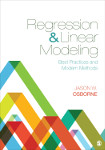# Regression & Linear Modeling: Best Practices and Modern Methods

In a conversational tone, Regression & Linear Modeling provides conceptual, user-friendly coverage of the generalized linear model (GLM). Readers will become familiar with applications of ordinary least squares (OLS) regression, binary and multinomial logistic regression, ordinal regression, Poisson regression, and loglinear models. The author returns to certain themes throughout the text, such as testing assumptions, examining data quality, and, where appropriate, nonlinear and non-additive effects modeled within different types of linear models.

•••